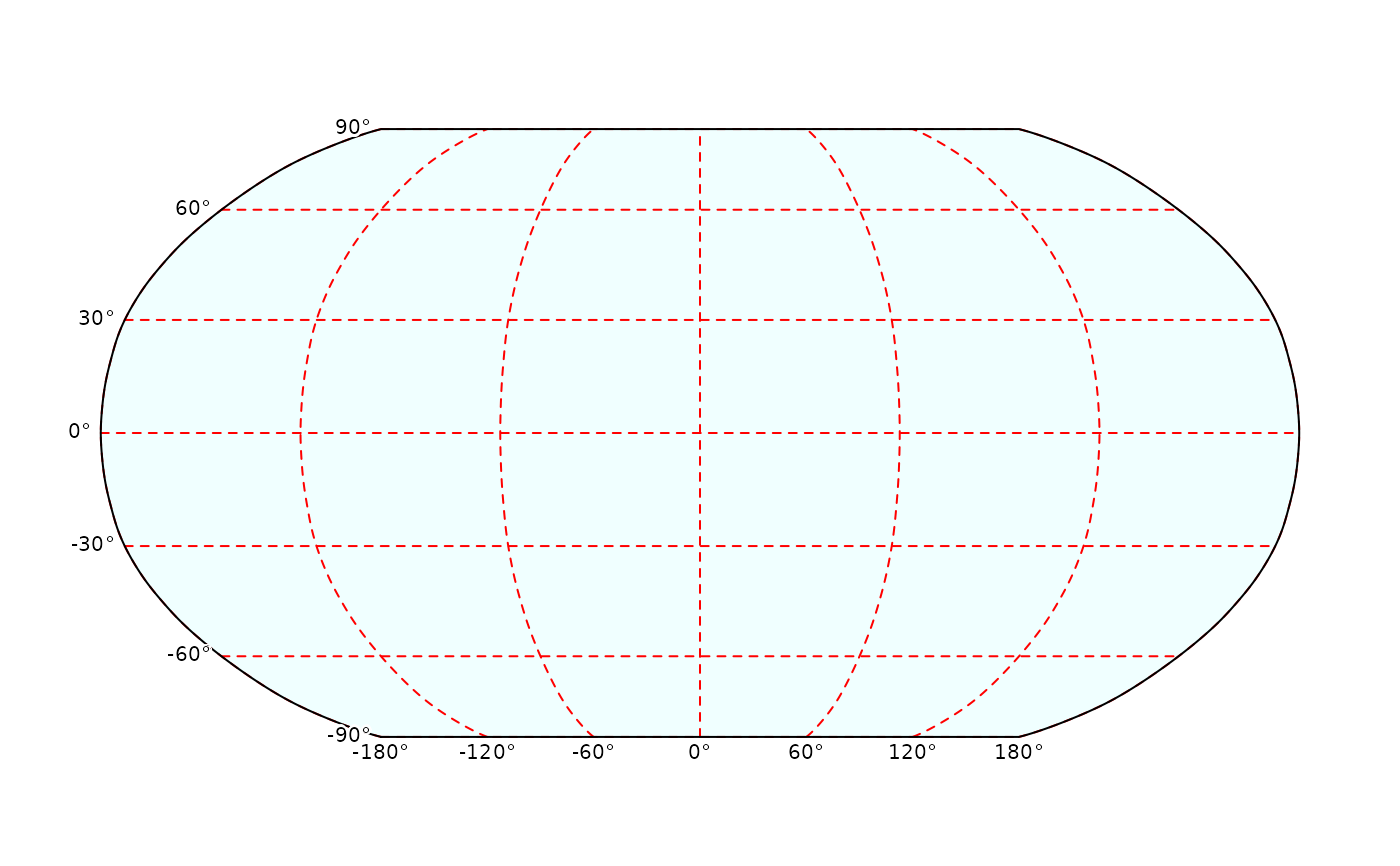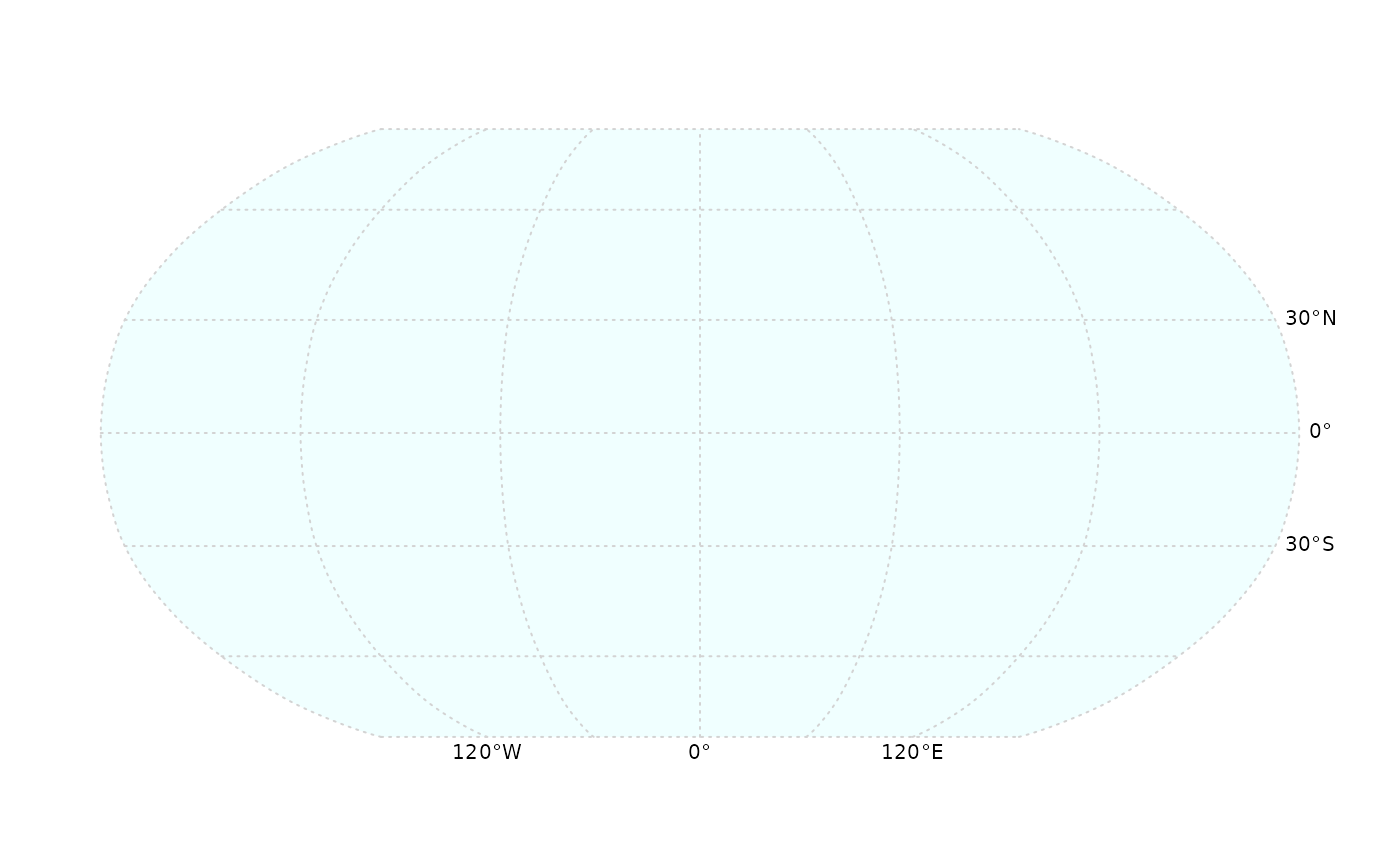Plot a SpatGraticule. You can create a SpatGraticule with graticule.

# S4 method for SpatGraticule,missing
plot(x, y, background=NULL, col="black", mar=NULL, labels=TRUE,
retro=FALSE, lab.loc=c(1,1), lab.lon=NULL, lab.lat=NULL, lab.cex=0.65,
lab.col="black", off.lat=0.25, off.lon=0.25, box=FALSE, box.col="black",
add=FALSE, ...)

## Arguments

x

SpatRaster or SpatVector

y

missing or positive integer or name indicating the layer(s) to be plotted

background

background color. If NULL, no background is drawn

mar

numeric vector of length 4 to set the margins of the plot. To make space for the legend you may use something like c(3.1, 3.1, 2.1, 7.1). To fill the plotting canvas, you can use c(0,0,0,0. Use NA to not set the margins

col

character. Color for the graticule lines

labels

logical. If TRUE, show graticule labels

retro

logical. If TRUE, show "retro" instead of decimal labels with the graticule

lab.loc

numeric. The first number indicates where the longitude graticule labels should be drawn (1=bottom, 2=top, NA=not drawn, any other number=top and bottom). The second number indicates where the latitude graticule labels should be drawn (1=left, 2=right, NA=not drawn, any other number=left and right)

lab.lon

positive integers between 1 and the number of labels, indicating which longitude graticule labels should be included

lab.lat

positive integers between 1 and the number of labels, indicating which latitude graticule labels should be included

lab.cex

double. size of the label font

lab.col

character. color of the labels

off.lon

numeric. longitude labels offset

off.lat

numeric. latitude labels offset

box

logical. If TRUE, the outer lines of the graticule are drawn on top with a sold line lty=1

box.col

character. color of the outer lines of the graticule if box=TRUE

logical. Add the graticule to the current plot?

...

additional graphical arguments passed to lines

graticule, plot, points, lines, polys, image, scatterplot, scale bar: sbar, north arrow: north
g <- graticule(60, 30, crs="+proj=robin")